Solve Linear Equations with Rational Numbers

Solve Linear Equations

(with Rational Numbers)

When solving linear equations, the goal is to isolate the unknown (variable) to find its value. This is accomplished by adding, subtracting, multiplying, or dividing.  Let’s start with a simple example:

x + 4 = 9

The main rule to keep in mind when trying to isolate a variable is that you always have to keep the equation balanced.  This means that whatever you do to one side of the equation, you must also do to the other side.  In the equation above, we need to get rid of the "+4" on the left side of the equation. We do this by using the opposite operation, which is subtraction.  So we subtract 4, which cancels out the "+4."  The x is now isolated, but we must also subtract 4 from the right side:

x + 4 = 9

- 4   - 4

x       = 5

For a more complicated equation, there might be more steps.  Let us take the example below:

3x - 9 = x + 3

When you have multiple operations involved in a problem, you must always do addition and subtraction first. Multiplication and division come second. You have a few options for what you do first with this problem.  Let us combine the x terms first.  We can subtract x from each side to get:

3x - 9 = x + 3

- x        - x

2x - 9 =    3

Then we will need to add 9 to each side:

2x - 9 = 3

+ 9   + 9

2x     = 12

Finally we need to isolate x.  2x is essentially 2 times x.  To cancel this out, we use the opposite operation, which is division.  We do this by dividing by 2.

2x = 12
2      2

x  =  6

You can always check your solution be plugging it back into the original equation.

3x + 9 = x + 3

3(6) + 9 = 6 + 3

18 + 9 = 9

9 = 9

Example 1: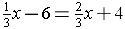First add 6 to each side to get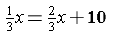Then you need to get rid of the fractions by multiplying each side by 3.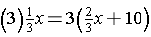x =  2x + 30

-2x    -2x

-x =          30

To make the x positive, we multiply each side by -1.  So the answer becomes

x = -30

Example 2:

7y + 5 - y + 1 = 2y - 6

This equation has like terms on one side of the equation.  You should combine them before beginning to isolate the variable.  So the equation becomes

6y + 6 = 2y - 6

- 6          - 6

6y       = 2y - 12

Then subtract 2y from each side and then divide both sides by 4:

4y  =  -12
4          4

y    =  -3

Practice Problems:

1.)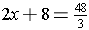2.)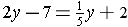3.)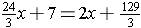4.)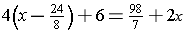1.) x = 4

2.) y = 5

3.) x = 6

4.) x = 10